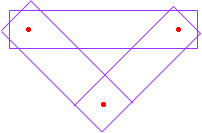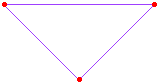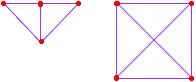Let us suppose some companies have collaborated to place several satellites in orbit. Let us call the set of all satellites that a given company helped place in orbit a network. Finally let us assume the following 4 rules. There are at least two distinct satellites. For each pair of satellites there is exactly one network containing them. Each network contains at least two distinct satellites. For each network, there is a satellite not in it. Draw a sketch using rectanges to represent networks and letters to represent satellites to illustrate the rules. What is the least number of satellites. what is the least number of networks? Thanks so much for the time and effort in helping me. David Hi David, By 1 there must be at least two satelites. If there are exactly two then by 2 there is a network containing them. But then 4 requires that there is another satellite. Hence there must be at least three satellites. Suppose that there are exactly three satellites. They can't all be in one netword or 4 is voilated. By 2 there is a network containing each pair of satellites so you cound try an arrangement as in the diagram.Here the red dots are the satellites and the rectangles are the networks. You should check to see that this arrangement satesfies the four conditions above. This is an example of a type of geometric structure called a finite linear space. The only difference is in the terminology. I would say points for satellites and lines for networks. The particular linear space you have above I would draw asThis is the only linear space with three points. There are two linear spaces with four points. They are illustrated below.Can you construct the four possible linear spaces with five points? Cheers, Penny Go to Math Central